# Math in Focus Grade 5 Chapter 9 Practice 4 Answer Key Dividing by Tens, Hundreds, and Thousands

Practice the problems of Math in Focus Grade 5 Workbook Answer Key Chapter 9 Practice 4 Dividing by Tens, Hundreds, and Thousands to score better marks in the exam.

## Math in Focus Grade 5 Chapter 9 Practice 4 Answer Key Dividing by Tens, Hundreds, and Thousands

Complete. Draw chips and use arrows to show how the chips move. Then fill in the blanks.

Question 1.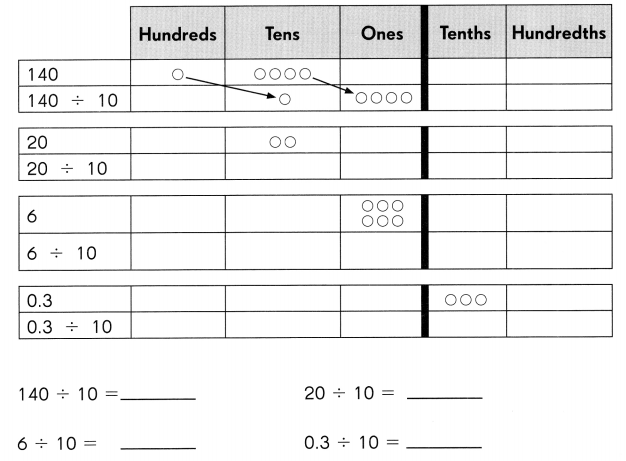140 ÷ 10 = 14
20 ÷ 10 = 2
6 ÷ 10 = 0.6
0.3 ÷ 10 = 0.03
Explanation:
When dividing by ten, move the decimal point one place to the left.
Place value is the value of a digit based on its location in the number.
When dividing by 100, move the decimal place two places to the left.

Divide.

Question 2.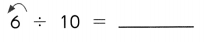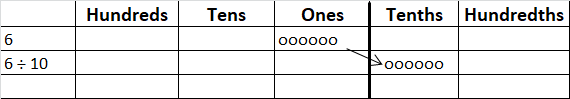Explanation:
When dividing by ten, move the decimal point one place to the left.
Place value is the value of a digit based on its location in the number.
When dividing by 100, move the decimal place two places to the left.
So, 6 is written as 0.6.

Question 3.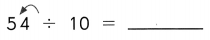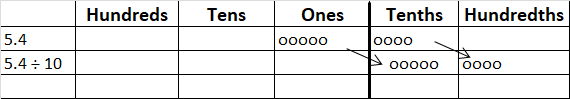Explanation:
When dividing by ten, move the decimal point one place to the left.
Place value is the value of a digit based on its location in the number.
When dividing by 100, move the decimal place two places to the left.
So, 5.4 is written as 0.54.

Question 4.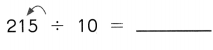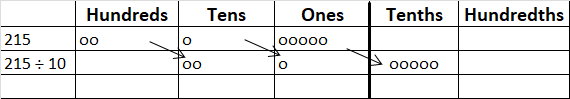Explanation:
When dividing by ten, move the decimal point one place to the left.
Place value is the value of a digit based on its location in the number.
When dividing by 100, move the decimal place two places to the left.
So, 215 will be come 21.5.

Question 5.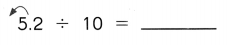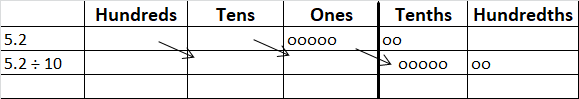Explanation:
When dividing by ten, move the decimal point one place to the left.
Place value is the value of a digit based on its location in the number.
When dividing by 100, move the decimal place two places to the left.
So, 5.2 can be written as 0.52.

Question 6.
64.6 ÷ 10 = ______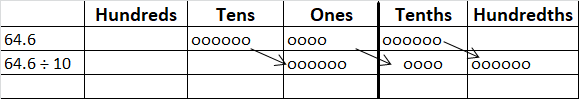Explanation:
When dividing by ten, move the decimal point one place to the left.
Place value is the value of a digit based on its location in the number.
So, 64.6 can be written as 6.46.

Question 7.
4.08 ÷ 10 = ______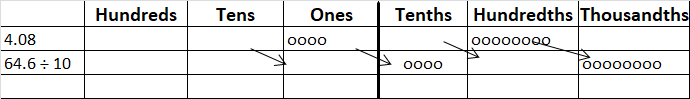Explanation:
When dividing by ten, move the decimal point one place to the left.
Place value is the value of a digit based on its location in the number.
So, 4.08 can be written as 0.408.

Question 8.
180.4 ÷ 10 = ______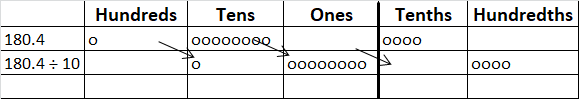Explanation:
When dividing by ten, move the decimal point one place to the left.
Place value is the value of a digit based on its location in the number,
after dividing by 10 , 180.4 is written as 18.04.

Question 9.
1.84 ÷ 1o = ______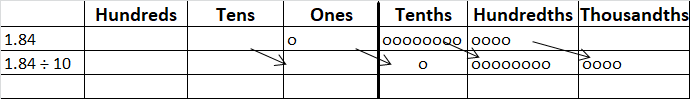Explanation:
When dividing by ten, move the decimal point one place to the left.
Place value is the value of a digit based on its location in the number,
after dividing by 10 , 1.84 is written as 0.184.

Complete.

Question 10.
23.7 ÷ ___ = 2.37
23.7 ÷ 10 = 2.37
Explanation:
When dividing by ten, move the decimal point one place to the left.
Place value is the value of a digit based on its location in the number,
after dividing by 10 , 23.7 is written as 2.37.

Question 11.
0.78 ÷ __ = 0.078
0.78 ÷ 10 = 0.078
Explanation:
When dividing by ten, move the decimal point one place to the left.
Place value is the value of a digit based on its location in the number,
after dividing by 10 , 0.78 is written as 0.078.

Question 12.
____ ÷ 10 = 4.106
41.06 ÷ 10 = 4.106
Explanation:
When dividing by ten, move the decimal point one place to the left.
Place value is the value of a digit based on its location in the number,
after dividing by 10 , 41.06 is written as 4.106.

Question 13.
___ ÷ 10 = 6.4
64 ÷ 10 = 6.4
Explanation:
When dividing by ten, move the decimal point one place to the left.
Place value is the value of a digit based on its location in the number,
after dividing by 10 , 64 is written as 6.4.

Divide

Example
9 ÷ 30 = (9 ÷ 3) ÷ 10
= 3 ÷ 10
= 0.3
So, 9 ÷ 30 = 0.3

Question 14.
0.09 ÷ 30 = (0.09 ÷ ___) ÷ 10
= ___ ÷ 10
= ______
So, 0.09 ÷ 30 = __
0.09 ÷ 30 = (0.09 ÷ 3) ÷ 10
= 0.03 ÷ 10
= 0.003
So, 0.09 ÷ 30 = 0.003
Explanation:
When dividing by ten, move the decimal point one place to the left.
Place value is the value of a digit based on its location in the number,
after dividing by 10.

Question 15.
0.09 ÷ 30 = (0.09 ÷ ___) ÷ 10
= ___ ÷ 10
= ______
So, 0.09 ÷ 30 = ____
0.09 ÷ 30 = (0.09 ÷ 3) ÷ 10
= 0.03 ÷ 10
= 0.003
So, 0.09 ÷ 30 = 0.003
Explanation:
When dividing by ten, move the decimal point one place to the left.
Place value is the value of a digit based on its location in the number,
after dividing by 10.

Question 16.
1.8 ÷ 90 = (1.8 ÷ ___ ) ÷ 10
= ___ ÷ 10
= ____
So, 1.8 ÷ 90 = ____
1.8 ÷ 90 = (1.8 ÷ 9 ) ÷ 10
= 0.2 ÷ 10
= 0.02
So, 1.8 ÷ 90 = 0.02
Explanation:
When dividing by ten, move the decimal point one place to the left.
Place value is the value of a digit based on its location in the number,
after dividing by 10.

Divide.

Question 17.
4.8 ÷ 20 = ___
Explanation:
4.8 ÷ 20 = (4.8 ÷ 2 ) ÷ 10
= 2.4 ÷ 10
= 0.24
So, 4.8 ÷ 20 = 0.24
Question 18.
0.32 ÷ 40 = ___
Explanation:
0.32 ÷ 40 = (0.32 ÷ 4 ) ÷ 10
= 0.08 ÷ 10
= 0.008
So, 0.32 ÷ 40 = 0.008
Question 19.
2.08 ÷ 80 = ___
Explanation:
2.08 ÷ 80 = (2.08 ÷ 8 ) ÷ 10
= 0.26 ÷ 10
= 0.026
So, 2.08 ÷ 80 = 0.026
Question 20.
2.55 ÷ 50 = ____
Explanation:
2.55 ÷ 50 = (2.55 ÷ 5 ) ÷ 10
= 0.51 ÷ 10
= 0.051
So, 2.55 ÷ 50 = 0.051
Question 21.
3.5 ÷ 70 = __
Explanation:
3.5 ÷ 70 = (3.5 ÷ 7 ) ÷ 10
= 0.5 ÷ 10
= 0.05
So, 3.5 ÷ 70 = 0.05
Question 22.
0.3 ÷ 60 = ___
Explanation:
0.3 ÷ 60 = (0.3 ÷ 6 ) ÷ 10
= 0.05 ÷ 10
= 0.005
So, 0.3 ÷ 60 = 0.005
Divide.

Question 23.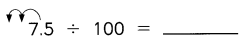Explanation:
7.5 ÷ 100 = (7.5 ÷ 10 ) ÷ 10
= 0.75 ÷ 10
= 0.075
So,  7.5 ÷ 100 = 0.075
When dividing by ten, move the decimal point one place to the left.
Place value is the value of a digit based on its location in the number.
When dividing by 100, move the decimal place two places to the left.

Question 24.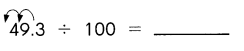Explanation:
49.3 ÷ 100 = (49.3 ÷ 10 ) ÷ 10
= 4.93 ÷ 10
= 0.493
So,  49.3 ÷ 100 = 0.493
Question 25.
6,000 ÷ 100 = ___
Explanation:
6000 ÷ 100 = (6000 ÷ 10 ) ÷ 10
= 600 ÷ 10
= 60
So,  6000 ÷ 100 = 60
Question 26.
708.2 ÷ 100 = ___
Explanation:
708.2 ÷ 100 = (708.2 ÷ 10 ) ÷ 10
= 70.82 ÷ 10
= 7.082
So,  708.2 ÷ 100 = 7.082
Question 27.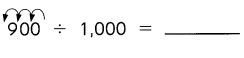Expalnation:
900 ÷ 1000 = (900 ÷ 10 ) ÷ 10 ÷ 10
= 90 ÷ 10÷ 10
= 9÷ 10
=0.9
So, 9000 ÷ 1000 = 0.9
Question 28.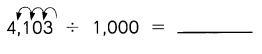Explanation:
4103 ÷ 1000 = (4103 ÷ 10 ) ÷ 10 ÷ 10
= 410.3 ÷ 10÷ 10
=41.03 ÷ 10
=4.103
So, 9000 ÷ 1000 = 4.103

Question 29.
909 ÷ 1,000 = ___
Explanation:
909 ÷ 1000 = (909 ÷ 10 ) ÷ 10 ÷ 10
= 90.9 ÷ 10÷ 10
= 9.09÷ 10
=0.909
So, 909 ÷ 1000 = 0.909

Question 30.
9,009 ÷ 1,000 = _______
Explanation:
9009 ÷ 1000 = (9009 ÷ 10 ) ÷ 10 ÷ 10
= 900.9 ÷ 10÷ 10
= 90.09÷ 10
=9.009
So, 9009 ÷ 1000 = 9.009

Complete.

Question 31.
86.2 ÷ _______ = 0.862
Explanation:
86.2 ÷ 100 = 0.862
When dividing by ten, move the decimal point one place to the left.
Place value is the value of a digit based on its location in the number.
When dividing by 100, move the decimal place two places to the left.

Question 32.
275 ÷ _______ = 0.275
Explanation:
275 ÷1000 = 0.275
When dividing by ten, move the decimal point one place to the left.
Place value is the value of a digit based on its location in the number.
When dividing by 100, move the decimal place two places to the left.

Question 33.
______ ÷ 100 = 0.006
Explanation:
0.6 ÷ 100 = 0.006
When dividing by ten, move the decimal point one place to the left.
Place value is the value of a digit based on its location in the number.
When dividing by 100, move the decimal place two places to the left.

Question 34.
______ ÷ 1,000 = 3.082
Explanation:
3082 ÷ 1,000 = 3.082
When dividing by ten, move the decimal point one place to the left.
Place value is the value of a digit based on its location in the number.
When dividing by 100, move the decimal place two places to the left.

Complete.

Example
0.07 = 0.7 ÷ 10
= 7 ÷ 100
= 70 ÷ 1,000

Question 35.
0.31 = 3.1 ÷ ______
= 31 ÷ ___
= 310 ÷ ____
0.31 = 3.1 ÷ 10
= 31 ÷ 100
= 310 ÷ 1000
Explanation:
When dividing by ten, move the decimal point one place to the left.
Place value is the value of a digit based on its location in the number.
When dividing by 100, move the decimal place two places to the left.
When dividing by 1000, move the decimal place three places to the left.

Question 36.
8.06 = ___ ÷ 10
= 806 ÷ ____
= 8,060 ÷ ___
8.06 = 80.6÷ 10
= 806 ÷ 100
= 8,060 ÷ 1000
Explanation:
When dividing by ten, move the decimal point one place to the left.
Place value is the value of a digit based on its location in the number.
When dividing by 100, move the decimal place two places to the left.
When dividing by 1000, move the decimal place three places to the left.

Question 37.
5.115 = ______ ÷ 10
= ___ ÷ 100
= 5,115 ÷ ______
5.115 = 51.15 ÷ 10
= 511.5 ÷ 100
= 5,115 ÷ 1000
Explanation:
When dividing by ten, move the decimal point one place to the left.
Place value is the value of a digit based on its location in the number.
When dividing by 100, move the decimal place two places to the left.
When dividing by 1000, move the decimal place three places to the left.

Complete.

Example
42 ÷ 200 = (42 ÷ 2) ÷ 100
= 21 ÷ 100
= 0.21
So, 42 ÷ 200 = 0.21

Question 38.
18.9 ÷ 900 = (18.9 ÷ ___) ÷ 100
= ___ ÷ 100
= ____
So, 18.9 ÷ 900 = ____
18.9 ÷ 900 = (18.9 ÷ 9) ÷ 100
= 2.1 ÷ 100
= 0.021
So, 18.9 ÷ 900 = 0.021
Explanation:
When dividing by ten, move the decimal point one place to the left.
Place value is the value of a digit based on its location in the number.
When dividing by 100, move the decimal place two places to the left.
When dividing by 1000, move the decimal place three places to the left.

Question 39.
2 ÷ 2,000 = (2 ÷ ___) ÷ 1,000
= ____ ÷ 1,000
= ____
So, 2 ÷ 2,000 = ____
2 ÷ 2,000 = (2 ÷ 2) ÷ 1,000
= 1 ÷ 1,000
= 0.001
So, 2 ÷ 2,000 = 0.001
Explanation:
When dividing by ten, move the decimal point one place to the left.
Place value is the value of a digit based on its location in the number.
When dividing by 100, move the decimal place two places to the left.
When dividing by 1000, move the decimal place three places to the left.

Question 40.
1,500 ÷ 6,000 = (1,500 ÷ ___) ÷ 1,000
= ______ ÷ 1,000
= ____
So, 1,500 ÷ 6,000 = ____
1,500 ÷ 6,000 = (1,500 ÷ 6) ÷ 1,000
= 250 ÷ 1,000
= 0.25
So, 1,500 ÷ 6,000 = 0.25
Explanation:
When dividing by ten, move the decimal point one place to the left.
Place value is the value of a digit based on its location in the number.
When dividing by 100, move the decimal place two places to the left.
When dividing by 1000, move the decimal place three places to the left.

Divide.

Question 41.
306 ÷ 600 = _______
Explanation:
306 ÷ 600 = (306 ÷ 6) ÷ 100
= 51 ÷ 100
= 0.51
Question 42.
29.7 ÷ 900 = ___
Explanation:
29.7 ÷ 900 = (29.7 ÷ 9) ÷ 100
= 3.3 ÷ 100
= 0.033
Question 43.
1.056 ÷ 800 = _______
Explanation:
1.056 ÷ 800 = (1.056 ÷ 8) ÷ 100
= 0.132 ÷ 100
= 0.00132
Question 44.
48 ÷ 2,000 = _______
Explanation:
48 ÷ 2000 = (48 ÷ 2 ) ÷ 10 ÷ 10 ÷ 10
= (24 ÷ 10) ÷ 10÷ 10
= (2.4 ÷ 10) ÷ 10
= (0.24 ÷ 10)
=0.024
So, 48 ÷ 2000 = 0.024
Question 45.
408 ÷ 3,000 = _______
Explanation:
408 ÷ 3000 = (408 ÷ 3 ) ÷ 10 ÷ 10 ÷ 10
= (136 ÷ 10) ÷ 10÷ 10
= (13.6 ÷ 10) ÷ 10
= (1.36 ÷ 10)
=0.136
So, 408 ÷ 3000 = 0.136
Question 46.
805 ÷ 7,000 = _______